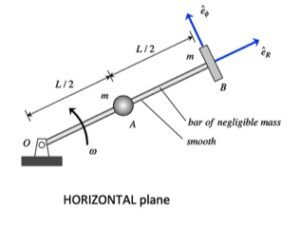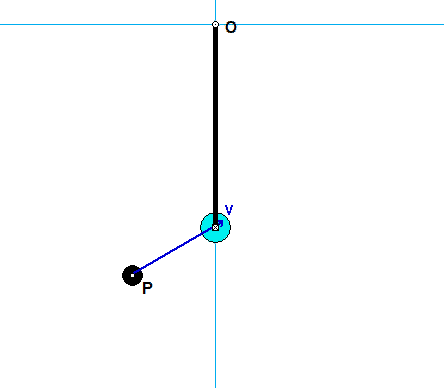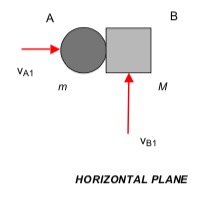# Homework H.4.S# Homework H.4.TDiscussionYou are asked to investigate the dynamics of this system during the short time of impact of P with A.

• It is suggested that you consider a system made up of A+P+bar (make the system "big").
• Draw a free body diagram (FBD) of this system.
• For this system, linear momentum is NOT conserved since there are non-zero reaction forces at O.
• Furthermore, energy is NOT conserved since there is an impact of P with A during that time.
• From your FBD of the system, you see that the moment about the fixed point O is zero. What does this say about the angular momentum of the system about O during impact? (Answer: It is conserved!)

HINTS:

STEP 1 - FBD: Draw a SINGLE free body diagram (FBD) of the system of A+P+bar.
STEP 2 - Kinetics:  Consider the discussion above in regard to conservation of angular momentum about point O. Recall how to calculate the angular momentum about a point for a particle.
STEP 3 - Kinematics: At Instant 2, the P sticks to A: vP2 = vA2.
STEP 4 - Solve.

# Homework H.4.QHINT: This problem is an exercise in becoming familiar with calculating angular momenta. Also, you will observe a lot of cancelation in the calculations with you sum together the contributions from the seven particles. Are you able to understand why these contributions occur?

# Homework H.4.RHINTS:
STEP 1 - FBD: The free body diagram of particle P shows only one force (the cable tension force), and this force always acts through the fixed point O.
STEP 2 - KINETICS: Above, you saw that all forces on P acted through the fixed point O - what does this say about the moments about point O and the consequences on the angular momentum about point O?
STEP 3 - KINEMATICS: The velocity of P is best written in terms of polar components: v = R_dot*e_R + R*φ_dot*e_φ. As discussed in class, you will not be able to calculate R_dot from the angular momentum equation. Recognizing this, you need to go back to STEP 2, and add on the work-energy equation for P. Question: How do you calculate the work done on P by the force F?
STEP 4 - SOLVE: At this point, you have a sufficient number of equations to solve for the two unknowns of R_dot and φ_dot.

# Homework H.4.O# Homework H.4.PAnimationHINTS:

STEP 1 - FBD: Draw three free body diagrams (FBDs): A, B and A+B.
STEP 2 - Kinetics:  From the FBD of A, we know that it will continue to move along the same line of travel. In addition, the FBD of B shows that the t-component of velocity for B will remain unchanged, where the "t"-direction is tangent to the plane of contact of A and B. In the n-direction (perpendicular to the t-direction), you have both the linear-impulse equation and the coefficient of restitution equation.
STEP 3 - Kinematics
STEP 4 - Solve: Solve for M and from your two kinetics equations above.

COMMENT: This problem is posed in a somewhat "backward" way as compared to many problems in impact. Typically mass and COR parameters are given, and we solve for final velocities. Here we are given some information on the final velocities, and we are asked to find mass and COR values. You use the same equations for either type of problem, you just solve these equations in a different way.

# Homework H.4.MHINTS:

STEP 1 - FBD: Draw a SINGLE free body diagram (FBD) of the system of cart + cannon + cannonball.
STEP 2 - Kinetics:  Write down the impulse/momentum equation in the horizontal direction (x-direction) for the the system of cart + cannon + cannonball. Based on the above FBD, is the momentum conserved in the x-direction for that system?
STEP 3 - Kinematics
STEP 4 - Solve. Solve for the velocity of the cart + cannon.

QUESTION: The above analysis allows you to find the answer to the first part of the problem. Unfortunately, it is not useful for the find the answer to the second part of the problem where you want to find the force on the cart/cannon. What do you need to change in the analysis to find this force?

# Homework H.4.NDiscussionShown above is the motion of particle B as it moves through the circular slot in A.

• Consider an FBD of a system made up of A and B together. Since there are no forces acting in the horizontal direction (x-direction), then linear momentum for the system of A+B is conserved in the x-direction. We see evidence of this as we watch the motion above: as the velocity in the x-direction for B increases to the right, then the velocity in of A increases to the left.
• From the above FBD, we can also say that energy is conserved for the system of A+B since there are no external non-conservative forces acting on the system that do work. A consequence of conservation of both energy and linear momentum is that as B reaches its maximum height in the circular slot the total kinetic energy for the system goes to zero since both momentum and kinetic energy was initially zero. You can readily see this in the above animation.

HINTS:

STEP 1 - FBD: Draw a SINGLE free body diagram (FBD) of the system of A+B.
STEP 2 - Kinetics:  Based on the above FBD (and the discussion above), you can use conservation of both energy and linear momentum in the x-direction. Write down both of these equations for the system of A+B.
STEP 3 - Kinematics: At Position 2, the velocity of B is strictly in the x-direction; that is:  vB2 =  vB2x.
STEP 4 - Solve.

# Homework H.4.KDiscussionShown above is the motion of the system made up of blocks A and B, with the velocities of A and B shown in the plots at the top. A is initially moving to the right, and B is initially moving to the left. As A slides on B, friction is generated at the contact of A and B. This friction slows down the motion of A with respect to B to the point where A is no longer moving to the right, and instead A begins moving to the left. You are asked to find the mutual speeds of A and B when sticking occurs.

HINTS:

STEP 1 - FBD: Draw a SINGLE free body diagram (FBD) of the system including Blocks A and B. Note that for this choice of system, the friction force between A and B is internal.
STEP 2 - Kinetics:  From your FBD above, there are no external forces acting on the system in the horizontal direction. What is the implication of this in terms of the linear momentum of the system? (HINT: It says that the linear momentum is conserved for the system of A + B. This becomes your kinetics equation. Note that energy is NOT conserved for this problem due to friction.)
STEP 3 - Kinematics: Note that when slipping ceases between blocks A and B, we have vA2 = vB2.
STEP 4 - Solve. Your final answer should be in terms of vA1 and vB1.

QUESTIONS:

• Should the final answer depend on the coefficient of friction? Does yours?
• Could you have worked out the problem by using two systems: A and B, individually? [Answer: Yes, but it requires more effort since you need to deal with the force of friction.]

__________________________